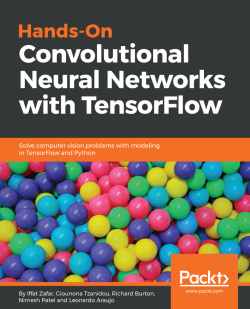### Hands-On Convolutional Neural Networks with TensorFlow4.7 (3 reviews total)
By Iffat Zafar , Giounona Tzanidou , Richard Burton and 2 more
• Start FREE trial Subscribe Access now
• \$23.99 eBook

• Constantly updated with 100+ new titles each month
• Breadth and depth in over 1,000+ technologies

Convolutional Neural Networks (CNN) are one of the most popular architectures used in computer vision apps. This book is an introduction to CNNs through solving real-world problems in deep learning while teaching you their implementation in popular Python library - TensorFlow. By the end of the book, you will be training CNNs in no time!

We start with an overview of popular machine learning and deep learning models, and then get you set up with a TensorFlow development environment. This environment is the basis for implementing and training deep learning models in later chapters. Then, you will use Convolutional Neural Networks to work on problems such as image classification, object detection, and semantic segmentation.

After that, you will use transfer learning to see how these models can solve other deep learning problems. You will also get a taste of implementing generative models such as autoencoders and generative adversarial networks.

Later on, you will see useful tips on machine learning best practices and troubleshooting. Finally, you will learn how to apply your models on large datasets of millions of images.

Publication date:
August 2018
Publisher
Packt
Pages
272
ISBN
9781789130331

## Chapter 1. Setup and Introduction to TensorFlow

TensorFlow is an open source software library created by Google that allows you to build and execute data flow graphs for numerical computation. In these graphs, every node represents some computation or function to be executed, and the graph edges connecting up nodes represent the data flowing between them. In TensorFlow, the data is multi-dimensional arrays called Tensors. Tensors flow around the graph, hence the name TensorFlow.

Machine learning (ML) models, such as convolutional neural networks, can be represented with these kinds of graphs, and this is exactly what TensorFlow was originally designed for.

In this chapter, we'll cover the following topics:

• Understanding the TensorFlow way of thinking
• Setting up and installing TensorFlow
• Introduction to TensorFlow API levels
• Building and training a linear classifier in TensorFlow
• Evaluating a trained model

## The TensorFlow way of thinking

Using TensorFlow requires a slightly different approach to programming than what you might be used to using, so let's explore what makes it different.

At their core, all TensorFlow programs have two main parts to them:

• Construction of a computational graph called `tf.Graph`
• Running the computational graph using `tf.Session`

In TensorFlow, a computational graph is a series of TensorFlow operations arranged into a graph structure. The TensorFlow graph contains two main types of components:

• Operations: More commonly called ops, for short, these are the nodes in your graph. Ops carry out any computation that needs to be done in your graph. Generally, they consume and produce Tensors. Some ops are special and can have certain side effects when they run.
• Tensors: These are the edges of your graph; they connect up the nodes and represent data that flows through it. Most TensorFlow ops will produce and consume these `tf.Tensors`.

In TensorFlow, the main object that you work with is called a Tensor. Tensors are the generalization of vectors and matrices. Even though vectors are one-dimensional and matrices are two-dimensional, a Tensor can be n-dimensional. TensorFlow represents Tensors as n-dimensional arrays of a user-specified data type, for example, `float32`.

TensorFlow programs work by first building a graph of computation. This graph will produce some `tf.Tensor` output. To evaluate this output, you must run it within a `tf.Session` by calling `tf.Session.run` on your output Tensor. When you do this, TensorFlow will execute all the parts of your graph that need to be executed in order to evaluate the `tf.Tensor` you asked it to run.

## Setting up and installing TensorFlow

TensorFlow is supported on the latest versions of Ubuntu and Windows. TensorFlow on Windows only supports the use of Python 3, while use on Ubuntu allows the use of both Python 2 and 3. We recommend using Python 3, and that is what we will use in this book for code examples.

There are several ways you can install TensorFlow on your system, and here we will go through two of the main ways. The easiest is by simply using the pip package manager. Issuing the following command from a terminal will install the CPU-only version of TensorFlow to your system Python:

`\$ pip3 install --upgrade tensorflow`

To install the version of Tensorflow that supports using your Nvidia GPU, simply type the following:

`\$ pip3 install --upgrade tensorflow-gpu`

One of the advantages of TensorFlow is that it allows you to write code that can run directly on your GPU. With a few exceptions, almost all the major operations in TensorFlow can be run on a GPU to accelerate their execution speed. We will see that this is going to be essential in order to train the large convolutional neural networks described later in this book.

### Conda environments

Using pip may be the quickest to get started, but I see that the most convenient method involves using conda environments.

Conda environments allow you to create isolated Python environments, which are completely separate from your system Python or any other Python programs. This way, there is no chance of your TensorFlow installation messing with anything already installed, and vice versa.

To use conda, you must download Anaconda from here: https://www.anaconda.com/download/. This will include conda with it. Once you've installed Anaconda, installing TensorFlow can be done by entering the certain commands in your Command Prompt. First, enter the following:

`\$ conda create -n tf_env pip python=3.5`

This will create your conda environment with the name `tf_env`, the environment will use Python 3.5, and `pip` will also be installed for us to use.

Once this environment is created, you can start using it by entering the following on Windows:

`\$ activate tf_env`

If you are using Ubuntu, enter the following command:

`\$ source activate tf_env`

It should now display `(tf_env)` next to your Command Prompt. To install TensorFlow, we simply do a pip install as previously, depending on if you want CPU only or you want GPU support:

`(tf_env)\$ pip install --upgrade tensorflow(tf_env)\$ pip install --upgrade tensorflow-gpu`

### Checking whether your installation works

Now that you have installed TensorFlow, let's check whether it works correctly. In your Command Prompt, activate your environment again if it isn't already, and run Python by entering the following:

`(tf_env)\$ python`

Now, enter the following lines into the Python interpreter to test that TensorFlow is installed correctly:

```>>>> import tensorflow as tf
>>>> x = tf.constant('Tensorflow works!')
>>>> sess = tf.Session()
>>>> sess.run(x)```

If everything is installed correctly, you should see the following output:

`b'Tensorflow works!'`

What you just typed there is the `Hello World` of TensorFlow. You created a graph containing a single `tf.constant`, which is just a constant Tensor. The Tensor was inferred to be of type string as you passed a string to it. You then created a TensorFlow Session, which is needed to run your graph and told your session to `run` on the Tensor that you created. The result of the Session running was then printed out. There is an extra `b` there because it's a byte stream that was created.

### Note

If you don't see the aforementioned and are getting some errors, your best bet is to check the following pages for solutions to common problems experienced when installing: Ubuntu: https://www.tensorflow.org/install/install_linux#common_installation_problems Windows: https://www.tensorflow.org/install/install_windows#common_installation_problems

## TensorFlow API levels

Before we get stuck into writing TensorFlow code, it is important to be aware of the different levels of API abstraction offered by TensorFlow in Python. This way, we can understand what is available to us when we write our code, and also we can choose the right functions or operations for the job. A lot of the time, there is little need to rewrite from scratch things that are already available for us to use in TensorFlow.

TensorFlow offers three layers of API abstraction to help write your code, and these can be visualized in the following diagram: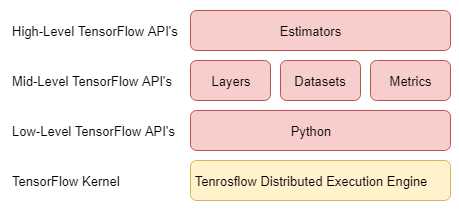At the lowest level, you have the basic TensorFlow ops such as `tf.nn.conv2d` and `tf.nn.relu`. These low-level primitives give the user the most control when working with TensorFlow. However, using them comes at the price of having to look after a lot more things yourself when constructing a graph and writing more boilerplate code.

Don't worry about understanding any of the following code examples yet, that will come very soon I promise; it is just here now for demonstrating the different API levels in TensorFlow.

So, for example, if we want to create a convolution layer to use in our ML model, then this might look something like the following:

```def my_conv_2d(input, weight_shape, num_filters, strides):
my_weights = tf.get_variable(name="weights", shape=weight_shape)
my_bias = tf.get_variable(name="bias", shape=num_filters)
my_conv = tf.nn.conv2d(input, my_weights, strides=strides, padding='same', name='conv_layer1')
conv_layer_out = tf.nn.relu(my_conv)
return conv_layer_out```

This example is much simpler than you would actually implement, but you can already see the number of lines of code starting to build up, along with things you have to take care of such as constructing weights and adding bias terms. A model would also have many different kinds of layers, not just a convolution layer, all having to be constructed in very similar ways to this.

So, not only is it quite laborious having to write these things out for every new kind of layer you want in your model, it also introduces more areas where bugs can potentially work their way into your code which is never a good thing.

Luckily for us, TensorFlow has a second level of abstraction that helps to make your life easier when building TensorFlow graphs. One example from this level of abstraction is the layers API. The layers API allows you to work easily with many of the building blocks that are common across many machine learning tasks.

The layers API works by wrapping up everything we wrote in the previous example and abstracting it away from us, so we don't have to worry about it anymore. For example, we can condense the preceding code to construct a convolution layer into one function call. Building the same convolution layer as before would now look like this:

```def my_conv_2d(input, kernel_size, num_filters, strides):
conv_layer_out = tf.layers.conv2d(input, filters=num_filters, kernel_size=kernel_size, strides=strides, padding='same', activation=tf.nn.relu, name='conv_layer1')
return conv_layer_out```

There are two other APIs that work alongside layers. The first is the datasets API that provides easy loading and feeding of data to your TensorFlow graph. The second one is the metrics API that provides tools to test how well your trained machine learning models are doing. We will learn about all these later in the book.

There is one final layer to the API stack that is the highest level of abstraction that TensorFlow provides, and that is called the estimators API. In much the same way that using `tf.layers` took care of constructing weights and adding biases for an individual layer, the estimators API wraps up construction of many layers so that we can define a whole model, made up of multiple different layers, in one function call.

The use of the estimators API will not be covered in this book, but if the reader wishes to learn more about estimators there are some useful tutorials available on the TensorFlow website.

This book will focus on using the low-level APIs along with the layers, datasets, and metrics APIs to construct, train, and evaluate your own ML models. We believe that by getting hands-on with these lower-level APIs the reader will come out with a greater understanding of how TensorFlow works under the hood, and be better equipped to tackle a wide variety of future problems that might have to use these lower-level functions.

### Eager execution

At the time of this writing, Google had just introduced the eager execution API to TensorFlow. Eager Execution is TensorFlow's answer to another deep learning library called PyTorch. It allows you to bypass the usual TensorFlow way of working where you must first define a computational graph and then execute the graph to get a result. This is known as static graph computation. Instead, with Eager Execution, you can now create the so-called dynamic graphs that are defined on the fly as you run your program. This allows for a more traditional, imperative way of programming when using TensorFlow. Unfortunately, eager execution is still under development with some features still missing, and will not be featured in this book. More information on Eager Execution can be found at the TensorFlow website.

## Building your first TensorFlow model

Without further ado, let's get stuck in with building your first ML model in TensorFlow.

The problem we will tackle in this chapter is that of correctly identifying the species of Iris flower from four given feature values. This is a classic ML problem that is extremely easy to solve, but will provide us with a nice way to introduce the basics of constructing graphs, feeding data, and training an ML model in TensorFlow.

The Iris dataset is made up of 150 data points, and each data point has four corresponding features: length, petal width, sepal length, and sepal width, along with the target label. Our task is to build a model that can infer the target label of any iris given only these four features.

Let's start by loading in our data and processing it. TensorFlow has a built-in function to import this particular dataset for us, so let's go ahead and use that. As our dataset is only very small, it is practical to just load the whole dataset into memory; however, this is not recommended for larger datasets, and you will learn better ways of dealing with this issue in the coming chapters. This following code block will load our data for us, an explanation of it will follow.

```import tensorflow as tf
import numpy as np

# Set random seed for reproducibility.
np.random.seed(0)
num_elements = len(labels)

# Use shuffled indexing to shuffle dataset.
shuffled_indices = np.arange(len(labels))
np.random.shuffle(shuffled_indices)
shuffled_data = data[shuffled_indices]
shuffled_labels = labels[shuffled_indices]

# Transform labels into one hot vectors.
one_hot_labels = np.zeros([num_elements,3], dtype=int)
one_hot_labels[np.arange(num_elements), shuffled_labels] = 1

# Split data into training and testing sets.
train_data = shuffled_data[0:105]
train_labels = shuffled_labels[0:105]
test_data = shuffled_data[105:]
test_labels = shuffled_labels[105:] ```

Let's once again take a look at this code and see what we have done so far. After importing TensorFlow and Numpy, we load the whole dataset into memory. Our data consists of four numerical features that are represented as a vector. We have 150 total data points, so our data will be a matrix of shape 150 x 4, where each row represents a different datapoint and each column is a different feature. Each data point also has a target label associated with it, which is stored in a separate label vector.

Next, we shuffle the dataset; this is important to do, so that when we split it into training and test sets we have an even spread between both sets and don't end up with all of one type of data in one set.

### One-hot vectors

After shuffling, we do some preprocessing on the data labels. The labels loaded with the dataset is just a 150-length vector of integers representing which target class each datapoint belongs to, either 1, 2, or 3 in this case. When creating machine learning models, we like to transform our labels into a new form that is easier to work with by doing something called one-hot encoding.

Rather than a single number being the label for each datapoint, we use vectors instead. Each vector will be as long as the number of different target classes you have. So for example, if you have 5 target classes then each vector will have 5 elements; if you have 1,000 target classes then each vector will have 1,000 elements. Each column in the vectors represents one of our target classes and we can use binary values to identify what class the vector is the label for. This can be done by setting all values to 0 and putting a 1 in the column for the class we want the vector label to represent.

This is easily understood with an example. For labels in this particular problem, the transformed vectors will look like this:

```1 = [1,0,0]
2 = [0,1,0]
3 = [0,0,1] ```

### Splitting into training and test sets

Finally, we take part of our dataset and put it to one side. This is known as our test set and we will not touch it until after we have trained our model. This set is used to evaluate how well our trained model performs on new data that it hasn't seen before. There are many approaches to how you should split your data up into training and test sets, and we will go into detail about them all later in the book.

For now though, we'll do a simple 70:30 split, so we only use 70% of our total data to train our model and then test on the remaining 30%.

## Creating TensorFlow graphs

Now that our data is all set up, we can construct our model that will learn how to classify iris flowers. We'll construct one of the simplest machine learning models—a linear classifier, as follows: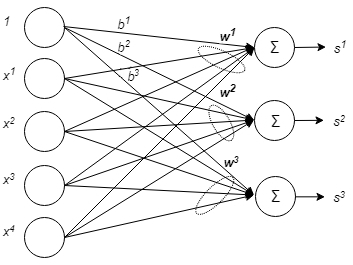A linear classifier works by calculating the dot product between an input feature vector x and a weight vector w. After calculating the dot product, we add a value to the result called a bias term b. In our case, we have three possible classes any input feature vector could belong to, so we need to compute three different dot products with w1, w2, and w3 to see which class it belongs to. But, rather than writing out three separate dot products, we can just do one matrix multiply between a matrix of weights of shape [3,4] and our input vector. In the following figure, we can see more clearly what it looks like: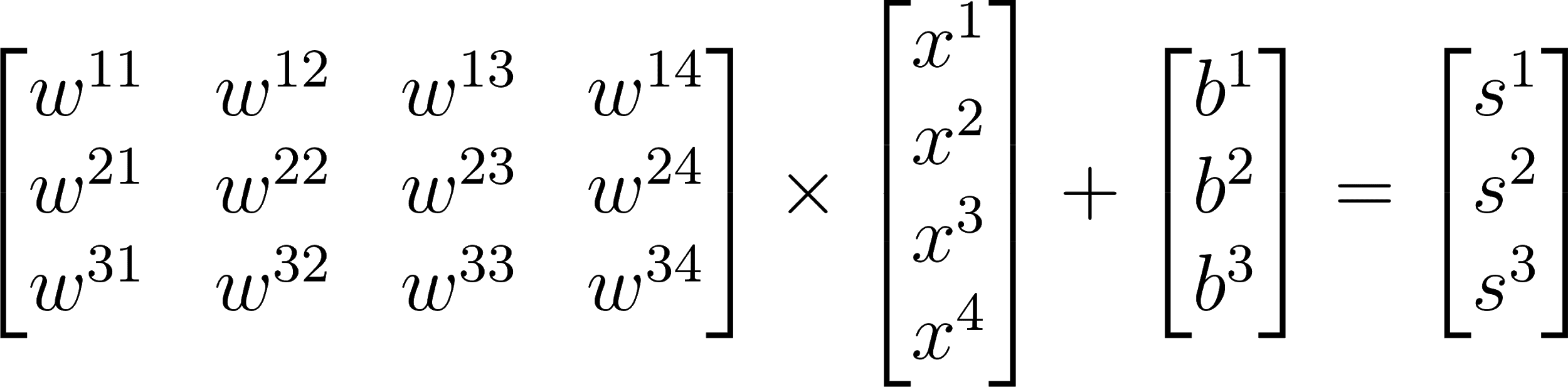We can also just simplify this equation down to the more compact form as follows, where our weight matrix is W, bias is b, x is our input feature vector and the resulting output is s: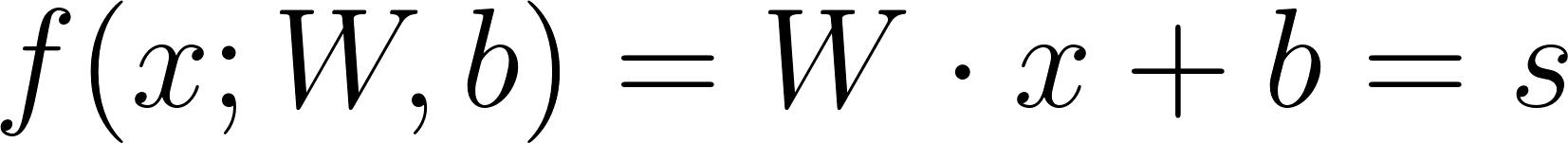### Variables

How do we write this all out in TensorFlow code? Let's start by creating our weights and biases. In TensorFlow, if we want to create some Tensors that can be manipulated by our code, then we need to use TensorFlow variables. TensorFlow variables are instances of the `tf.Variable` class. A `tf.Variable` class represents a `tf.Tensor` object that can have its values changed by running TensorFlow operations on it. Variables are Tensor-like objects, so they can be passed around in the same ways Tensors can and any operation that can be used with a Tensor can be used with a variable.

To create a variable, we can use `tf.get_variable()`. When you call this function, you must supply a name for your variable. This function will first check that there is no other variable with the same name already on the graph, and if there isn't, then it will create and add a new one to the TensorFlow graph.

You must also specify the shape that you want your variable to have, or alternatively, you can initialize your variable using a `tf.constant` Tensor. The variable will take the value of your constant Tensor and the shape will be automatically inferred. For example, the following will produce a 1x2 Tensor containing the values 21 and 25:

`my_variable = tf.get_variable(name= "my_variable", initializer=tf.constant([21, 25]))`

### Operations

It's all well and good having variables in our graph, but we also want to do something with them. We can use TensorFlow ops to manipulate our variables.

As explained, our linear classifier is just a matrix multiply so the first op you will use is funnily enough going to be the matrix multiply op. Simply call `tf.matmul()` on two Tensors you want to multiply together and the result will be the matrix multiplication of the two Tensors you passed in. Simple!

Throughout this book, you will learn about many different TensorFlow ops that you will need to use.

Now that you hopefully have a little understanding about variables and ops, let's construct our linear model. We'll define our model within a function. The function will take as input N lots of our feature vectors or to be more precise a batch of size N. As our feature vectors are of length 4, our batch will be an [N, 4] shape Tensor. The function will then return the output of our linear model. In the following code, we have written our linear model function, it should be self explanatory but keep reading if you have not completely understood it yet.

```def linear_model(input):
# Create variables for our weights and biases
my_weights = tf.get_variable(name="weights", shape=[4,3])
my_bias = tf.get_variable(name="bias", shape=)

# Create a linear classifier.
linear_layer = tf.matmul(input, my_weights)
return linear_layer_out ```

In the code here, we create variables that will store our weights and biases. We give them names and supply the required shapes. Remember we are using variables as we want to manipulate their values using operations.

Next, we create a `tf.matmul` node that takes as argument our input feature matrix and our weight matrix. The result of this op can be accessed through our `linear_layer` Python variable. This result is then passed to another op, `tf.nn.bias_add`. This op comes from the NN (neural network) module and is used when we wish to add a bias vector to the result of a calculation. A bias has to be a one-dimensional Tensor.

## Feeding data with placeholders

Placeholders are Tensor-like objects. They are a contract between you and TensorFlow that says when you run your computation graph in a session, you will supply or feed data into that placeholder so that your graph can run successfully.

They are Tensor-like objects as they behave like Tensors, meaning you can pass them around in places where you would put a Tensor.

By using placeholders, we can supply external inputs into our graph that might change each time we run our graph. The natural use for them is as a way to supply data and labels into our model as the data and labels we supply will generally be different each time we want to run our graph.

When creating a placeholder, we must supply the datatype that will be fed.

We will use two placeholders to supply data and labels into our graph. We also supply the shape that any data fed into these placeholders must take. We use `None` to indicate the size of that particular dimension can take any value. This way we are able to feed in batches of data that are varying sizes. Following we'll see how to define placeholders in TensorFlow for our problem.

```x = tf.placeholder(tf.float32, shape=[None, 4], name="data_in")
y = tf.placeholder(tf.int32, shape=[None, 3], name="target_labels") ```

Now, we have created placeholders in our graph, so we can construct our linear model on the graph as well. We call our function that we defined previously, and supply as input our data placeholder, `x`. Remember, placeholders act like Tensors so they can be passed around like them as well. In the following code we call our linear_model function with our placeholder as the input argument.

`model_out = linear_model(x)`

When we call our function, everything inside it executes and all the ops and variables are added to our TensorFlow graph. We only need to do this once; if we were to try calling our function again, we would get an error saying that we have tried to add variables to the graph but they already exist.

Placeholders are the simplest and quickest way of supplying external data into our graph, so it's good to know about them. Later on, we will see better ways of supplying data using the dataset API, but for now placeholders are a good place to start.

## Initializing variables

Before we are able to use our variables in our graph, we must initialize them. We need to create a graph node that will do this for us. Using `tf.global_variables_initializer` will add an initializer node to our graph. If we run this node in a session, then all the variables in our graph will become initialized so that we are able to use them. So, for now, let's create an initializer node as follows:

`initializer = tf.global_variables_initializer()`

As we did not explicitly say what kind of initialization to use for our variables, TensorFlow will use a default one called the Glorot Normal Initializer, which is also known as Xavier Initialization.

## Training our model

We have constructed the graph of our linear model, and we can supply data into it. If we were to create a session and run the `model_out` Tensor in it while supplying some input data, then we would get a result produced. However, the output we would get would be complete rubbish. Our model has yet to be trained! The values of our weights and biases just have the default values given to them when we initialized our variables using the initializer node.

### Loss functions

To train our model, we must define something called a loss function. The loss function will tell us how well or badly our model is currently doing its job.

Losses can be found in the `tf.losses` module. For this model, we will use the hinge loss. Hinge loss is the loss function used when creating a support vector machine (SVM). Hinge loss heavily punishes incorrect predictions. For one given example,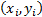, where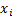is a feature vector of a datapoint and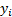is its label, the hinge loss for it will be as follows: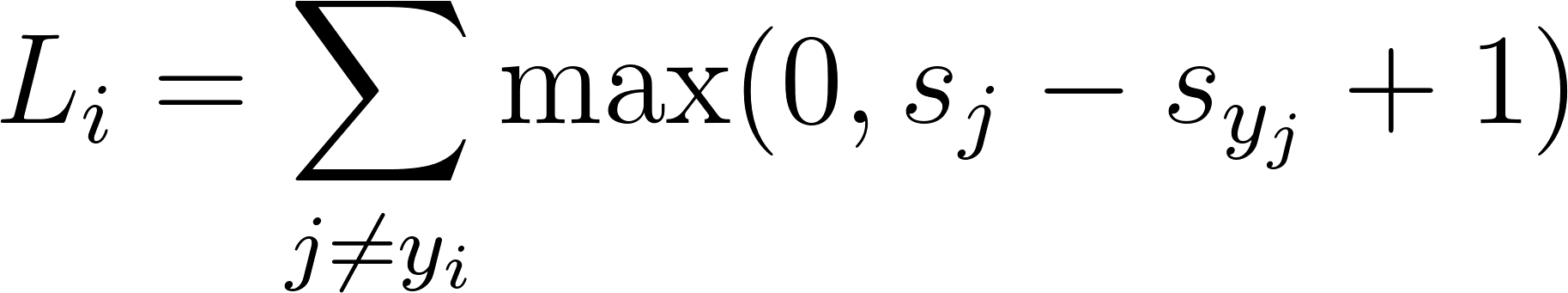To this, the following will apply: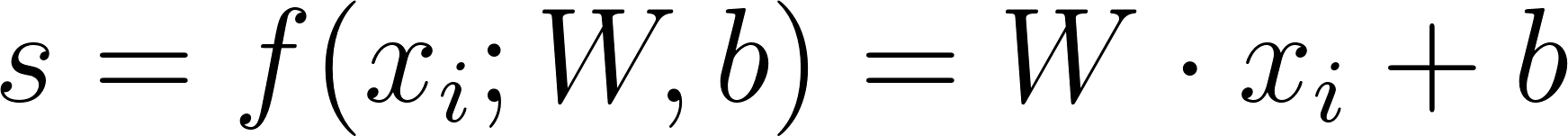In simple words, this equation takes the raw output of the classifier. In our model, that's three output scores, and ensures that the score of the target class is greater, by at least 1, than the scores of the other classes. For each score (except the target class), if this restriction is satisfied, then 0 is added to the loss, otherwise, there's a penalty that is added: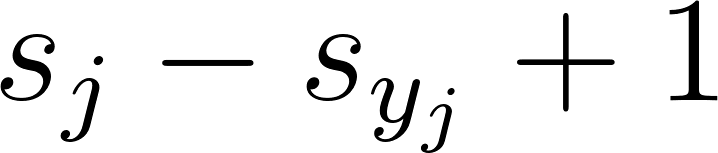This concept is actually very intuitive because if our weights and biases are trained properly, then the highest of the three produced scores should confidently indicate the correct class that an input example belongs to.

Since during training we feed many training examples in at once, we'll obtain multiple losses like these that need to be averaged. Therefore, the total loss equation that needs to be minimized is as follows: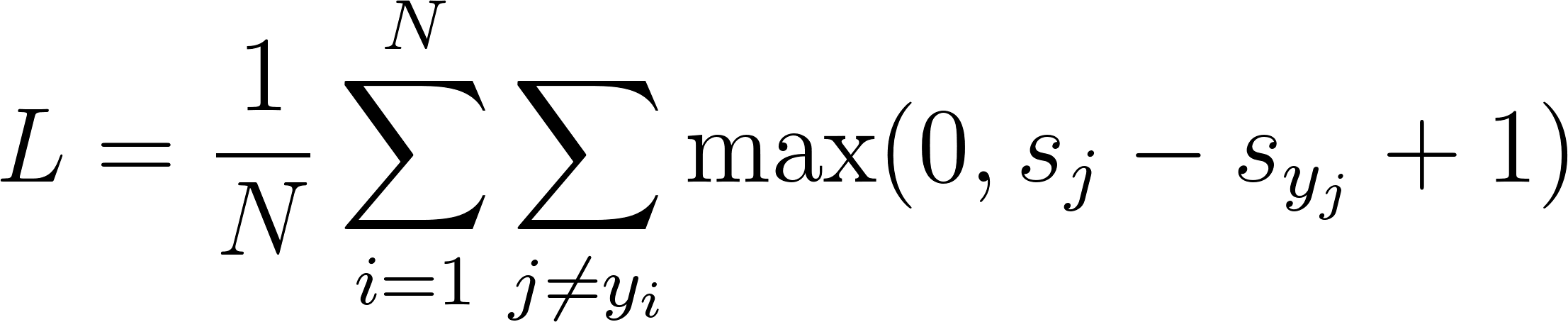In our code, the loss function will take two arguments: logits and labels. In TensorFlow, logits is the name for the raw values produced by our model. In our case, this is `model_out` as this is the output of our model. For labels, we use our label placeholder, `y`. Remember that the placeholder will be filled for us at runtime:

`loss = tf.reduce_mean(tf.losses.hinge_loss(logits=model_out, labels=y))`

As we also want to average our loss across the whole batch of input data, so we use `tf.reduce_mean` to average all our losses into one loss value that we will minimize.

There are many different types of lossfunctions available for us to use that are all good for different machine learning tasks. As we go through the book, we will learn more of them and when to use different loss functions.

### Optimization

Now we have defined a loss function to be used; we can use this loss function to train our model. As is shown in the previous equations, the loss function is a function of weights and biases. Therefore, all we have to do is an exhaustive search of the space of weights and biases and see which combination minimizes the loss best. When we have one- or two-dimensional weight vectors, this process might be okay, but when the weight vector space gets too big, we need a more efficient solution. To do this, we will use an optimization technique called gradient descent.

By using our loss function and calculus, gradient descent is able to see how to adjust the values of the weights and biases of our model in such a way that the value of the loss decreases. It is an iterative process requiring many iterations before the values of our weights and biases are well-adjusted for our training data. The idea is that the loss function L, parametrized by weights w, is minimized by updating the parameters in the opposite direction of the gradient of the objective function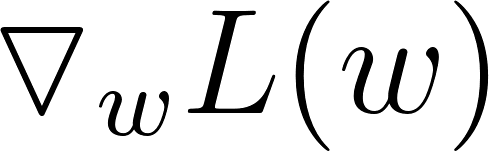with respect to the parameters. The update functions for weights and biases look like the following: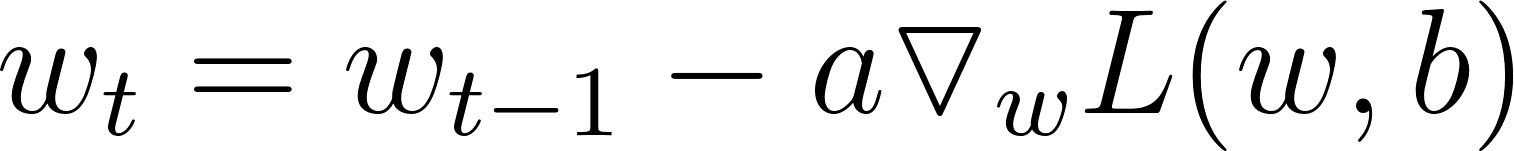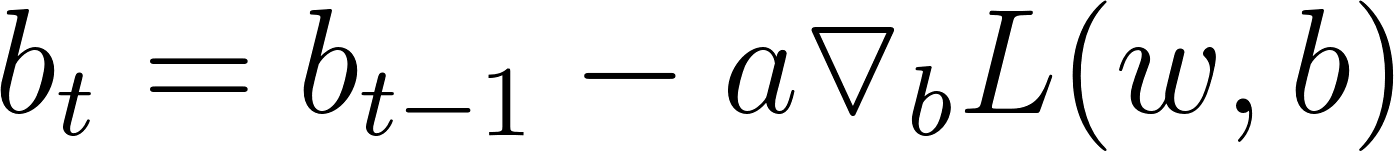Here,is the iteration number and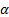is a hyperparameter called the learning rate.

A loss function that is parameterized by two variables w1 and w2 will look something like in the following diagram: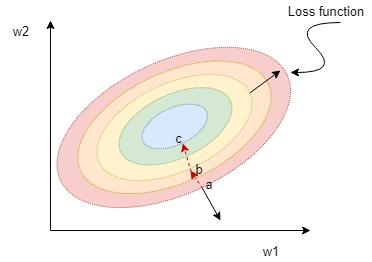The preceding diagram shows the level curves of an elliptical paraboloid. This is a bowl-shaped surface and the bottom of the bowl lies at the center. Looking at the plot, the gradient vector at point a (the straight black arrow) is normal to the level curve through a. The gradient vector, in fact, points in the direction of the greatest rate of increase of the loss function.

So, if we start from point a and update the weights toward the direction opposite to the gradient vector, then we will descend to point b and in the next iteration to point c, and so on until we reach the minimum. The parameters that minimize the loss function are selected to represent the final trained linear model.

The nice thing about TensorFlow is it calculates all the required gradients for us using its built-in optimizers with something called automatic differentiation. All we have to do is choose a gradient descent optimizer and tell it to minimize our loss function. TensorFlow will automatically calculate all the gradients and then use these to update our weights for us.

We can find optimizer classes in the `tf.train` module. For now, we will use the `GradientDescentOptimizer` class, which is just the basic gradient descent optimization algorithm. When creating the optimizer, we must supply a learning rate. The value of the learning rate is a `hyperparameter` that the user must tune through trial and error and experimentation. The value of 0.5 should work well in this problem.

The optimizer node has a method called `minimize`. Calling this method on a loss function that you supply will do two things. First, gradients with respect to this loss are calculated for your whole graph. Second, these gradients are used to update all relevant variables.

Creating our optimizer node will look something like this:

`optimizer = tf.train.GradientDescentOptimizer(learning_rate=0.5).minimize(loss) `

Like with loss functions, there are many different flavors of gradient descent optimizers to learn about. Presented here is the most basic kind, but again, we will learn about and use different ones in future chapters.

## Evaluating a trained model

We have put together all the pieces we need in order to train our model. The last thing before we start training is that we want to create some nodes in our graph that will allow us to test how good our model has done after we have finished training it.

We will create a node that calculates the accuracy of our model.

`Tf.equal` will return a Boolean list indicating where the two supplied lists are equal. Our two lists, in this case, will be the label and the output of our model, after finding the indices of the max values:

`correct_prediction = tf.equal(tf.argmax(model_out,1), tf.argmax(y,1)) `

We can then use `reduce_mean` again to get the average number of correct predictions. Don't forget to cast our `boolean correct_prediction` list back to `float32`:

`accuracy = tf.reduce_mean(tf.cast(correct_prediction, tf.float32)) `

## The session

Now we have constructed all the parts of our computational graph. The very final thing we need to do is create a `tf.Session` and run our graph. The TensorFlow session is a way to connect your TensorFlow program, written in Python, with the C++ runtime powering TensorFlow. The session also gives TensorFlow access to devices such as CPUs and GPUs present on your local or remote machine. In addition, the session will cache information about the constructed graph so computation can be efficiently run many times.

The standard way to create a session is to do so using a Python context manager: the `with` statement block:

`with tf.Session() as sess:.  `

The reason for this is that when you create a session, it has control of CPU, memory, and GPU resources on your computer. When you are finished using your session, you want all these resources to be freed up again, and the easiest way to ensure this is by using a `with` statement.

The first thing we'll do after creating our session is to run our initializer op. You can evaluate nodes and Tensors in a graph using a session by calling `tf.Session.run` on the graph objects you want to evaluate. When you supply part of your graph to `session.run`, TensorFlow will work its way through the graph evaluating everything that the supplied graph part depends on in order to produce a result.

So, in our example, calling `sess.run(initializer)` will search back through the graph, find everything that is required to execute the initializer, and then execute these nodes in order. In this case, nothing is connected to the initializer node, so it will simply execute this one node that initializes all our Variables.

Now that our variables are initialized, we start the training loop. We will train for 1000 steps or iterations, so we create a for loop where our training steps will take place. The amount of steps to train for is a `hyperparameter` of sorts; it is something that we need to decide on when we train our model. There can be trade-offs with the value you choose, and this will be discussed in the future chapters. For this problem, 1000 steps will be good enough to get the desired result.

We grab a batch of training data and labels that we will feed into our graph. Next, we call `session.run` again. This time, we call it on two things, the loss and optimizer. We can supply as many things as we want to evaluate by putting them in a list that we supply to `session.run`. TensorFlow will be smart enough not to evaluate the graph multiple times if it doesn't need to, and it will reuse results that have already been calculated. This list we supply is called our fetches; it is the nodes in the graph that we want to evaluate and fetch.

After the list of fetches, we supply a `feed_dict` or feed dictionary. This is a dictionary in which each key is the Tensor in the graph that we will feed values to (in this case, our placeholders) and the corresponding value is the value that will be fed to it.

The return values of `session.run` correspond to each of the values in our fetch list. Our first fetch is the loss Tensor in our graph, so the first return argument comes from this. The second fetch is the optimizer node. We don't care about what is returned from this node as we only care about what the optimizer node calculates, so we leave its corresponding return empty:

```with tf.Session() as sess:
sess.run(initializer)

for i in range(1000):
batch_x, batch_y = train_data[:,:], train_labels[:,:]

loss_val, _ = sess.run([loss, optimizer], feed_dict={x : batch_x, y: batch_y})
print("Train Accuracy:", sess.run(accuracy, feed_dict={x: train_data, y: train_labels}))
print("Test Accuracy:", sess.run(accuracy, feed_dict={x: test_data, y: test_labels})) ```

After running for 1000 iterations, we use another `session.run` call to fetch the output of our accuracy node. We do this twice, once feeding in our training data to get accuracy on the training set, and once feeding in our held out test data to get the accuracy on the test set. You should get a test accuracy printed out of `0.977778`, which means our model correctly classified 44 out of 45 of our test sets, not too bad at all!

## Summary

In this chapter, we have explained how programming with TensorFlow works and how to set up your work environment for working with TensorFlow. We have also looked at how to build, train, and evaluate your own linear model using TensorFlow for classifying iris flowers. In doing so, we briefly looked at loss functions and gradient descent optimizers.

In the next chapter, we will learn more about some key deep-learning concepts, including convolutional neural networks. We'll also look at how to use TensorFlow to build and train deep neural networks.

• ##### Iffat Zafar

Iffat Zafar was born in Pakistan. She received her Ph.D. from the Loughborough University in Computer Vision and Machine Learning in 2008. After her Ph.D. in 2008, she worked as research associate at the Department of Computer Science, Loughborough University, for about 4 years. She currently works in the industry as an AI engineer, researching and developing algorithms using Machine Learning and Deep Learning for object detection and general Deep Learning tasks for edge and cloud-based applications.

Browse publications by this author
• ##### Giounona Tzanidou

Giounona Tzanidou is a PhD in computer vision from Loughborough University, UK, where she developed algorithms for runtime surveillance video analytics. Then, she worked as a research fellow at Kingston University, London, on a project aiming at prediction detection and understanding of terrorist interest through intelligent video surveillance. She was also engaged in teaching computer vision and embedded systems modules at Loughborough University. Now an engineer, she investigates the application of deep learning techniques for object detection and recognition in videos.

Browse publications by this author
• ##### Richard Burton

Richard Burton graduated from the University of Leicester with a master's degree in mathematics. After graduating, he worked as a research engineer at the University of Leicester for a number of years, where he developed deep learning object detection models for their industrial partners. Now, he is working as a software engineer in the industry, where he continues to research the applications of deep learning in computer vision.

Browse publications by this author
• ##### Nimesh Patel

Nimesh Patel graduated from the University of Leicester with an MSc in applied computation and numerical modeling. During this time, a project collaboration with one of University of Leicester’s partners was undertaken, dealing with Machine Learning for Hand Gesture recognition. Since then, he has worked in the industry, researching Machine Learning for Computer Vision related tasks, such as Depth Estimation.

Browse publications by this author
• ##### Leonardo Araujo

Leonardo Araujo is just the regular, Brazilian, curious engineer, who has worked in the industry for the past 19 years (yes, in Brazil, people work before graduation), doing HW/SW development and research on the topics of control engineering and computer vision. For the past 6 years, he has focused more on Machine Learning methods. His passions are too many to put on the book.

Browse publications by this author

### Latest Reviews

(3 reviews total)